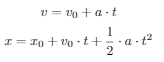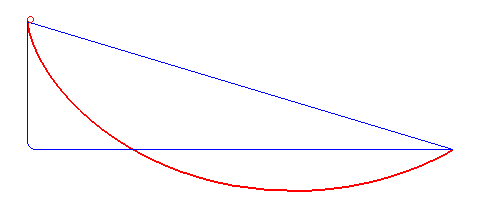top of page
Search

# An Introduction To The Brachistochrone Problem

Updated: Nov 4

Author: Satyam SharmaWhat is the fastest way to get from point A to point B, when viewing the problem two- dimensionally, where Point B is at a lesser height than Point A (but not directly below it)?

It’s well known that the shortest distance between two points on a piece of paper is a line, so it might be natural for one to assume that the fastest distance follows from the fact that:Logically speaking, the distance is minimized, and thus since distance is directly proportional to time, time must be minimized as well.However, the fallacy in this chain of thought occurs when something fundamental is realized: this equation only holds for constant velocity. Yet, we never put any such constraints when we posed our initial question; the distance between two points, a ramp, can be curved just as validly as it can be straight.Taking another logical approach, it follows that perhaps the curve with the greatest slope provides the greatest acceleration, and thus the greatest velocity. After all:which means that as acceleration increases, so does velocity, and as velocity increases, logically time decreases.

However, the fallacy in this chain of thought happens when something even deeper is realized:

The equation only holds for uniform/constant acceleration. In fact, what happens in the pictured curve is that the object, when dropped onto this ramp, accelerates rapidly, then accelerates slowly. So maximizing the initial acceleration, while seemingly an insightful path to follow, negates its own benefits due to the fact that the latter half of the ramp must meaningfully be flat (and thus with little to no acceleration).

We now have intuitively realized that the “ideal curve” must be somewhere in between ── steep enough to gain acceleration/velocity quickly, but not too steep, because then it will negate itself later on.

This shortest time problem has a name: the brachistochrone problem (‘brachistos’, Greek for shorter, and ‘chronos’, Greek for time) has been around for several centuries, and confused mathematicians, geometers, and physicists alike until Johann Bernoulli used Newton’s then new-found tool of calculus.

Bernoulli made a key assumption when tackling this problem: the curve is independent of the local strength of gravity as well as the mass of the test body. In his own words presented to the readers of the first scientific journal in the German language,

“Given two points A and B in a vertical plane, what is the curve traced out by a point acted on only by gravity, which starts at A and reaches B in the shortest time.”

With reference to these GIFs, the problem can be rephrased as: “is there a red ramp, such as the one shown in the GIFs, such that an object travelling along that ramp under only the influence of gravity can always reach the end point faster than any other alternative (blue) ramp?”

This post’s intention is to only serve as an introduction to the problem and showcase some errors in logical lines of thinking, so resources to the actual solution to the problem can be found in this amazing post by Mark Levi, this video by 3Blue1Brown, and this more analytical/mathematical derivation by KhanAcademy.This post’s intention is to only serve as an introduction to the problem and showcase some errors in logical lines of thinking. Resources to the actual solution to the problem can be found in this amazing post by Mark Levi, this video by 3Blue1Brown, and this more analytical/mathematical derivation by Khan Academy.

As a fun treat to play around with, here is a engageable demonstration about the nature of roulettes. Roulettes are the path traced by a rolling object, and the roulette traced by a point in, on the perimeter of, or outside the rolling object is called a trochoid.

When the point that is acting as the tracer object is on the circumference of a circle, and the circle is rolling on a single line (or a flat surface), the contour figure that remains is known as a cycloid. A cycloid can be found by setting the ratio in the interactive demonstration equal to one.

See if you can find, by inverting the resulting shapes you create in the demo, a brachistochrone. Have fun!